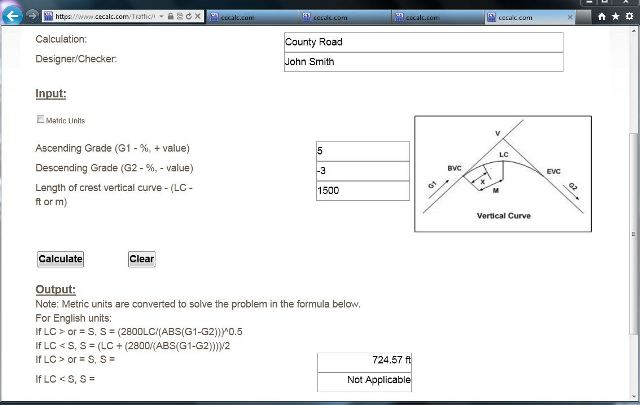## Transportation Design Problem 1 - Determine passing sight distance for a crest vertical curve:

Given a crest vertical curve connecting an ascending grade of 5% to a descending grade of 3%. If the PVC is at station 23+00 and the PVT is at station 38 + 00, what is the required passing sight distance.
##### 1.
From the transportation submenu open the passing sight distance crest vertical curve calculation, CrstVrtCrvPassS.aspx. Input the "Title" of the calculation, the "Designer/Checker" information and the variables.  LC = 3800 - 2300 = 1500 feet. Click on the "Calculate" button. Read the results. The minimum passing sight distance is 724.57 feet.##### 2. Summary
The minimum passing sight distance is 724.57 feet.

 Start Using The Calculations >>> Become A Member Now!